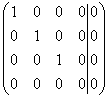# Strange matrix problem

Hi,

## Homework Statement

I was given a linear equation system of the form Ax=b where x=(x, y, z, w),
I reduced (A|b) to its canonical form which is this:

## Homework Equations## The Attempt at a Solution

At first I thought that this means that there is an infinite number of solutions with one freedom degree.
But I cannot imagine in the world what the solution vector might look like.
I am sorry if this seems like a stupid question but can anyone plz explain this to me?

Thanks

Mark44
Mentor
Hi,

## Homework Statement

I was given a linear equation system of the form Ax=b where x=(x, y, z, w),
I reduced (A|b) to its canonical form which is this:

## Homework Equations## The Attempt at a Solution

At first I thought that this means that there is an infinite number of solutions with one freedom degree.
But I cannot imagine in the world what the solution vector might look like.
I am sorry if this seems like a stupid question but can anyone plz explain this to me?

Thanks

Just read them off your final augmented matrix: x = 0, y = 0, z = 0; w is arbitrary. The solution represents the line in four-dimensional space that coincides with the w-axis.

Do you mean that if we assign w=t a general solution could be V=(0,0,0,t)?

Mark44
Mentor
Yes.

O.K Iunderstand the technical side of what you are saying Av=0, but the equation you get is 0w=0. How can this have any meaning?

Mark44
Mentor
Of course the equation 0w = 0 has meaning. It's true for any value of w.

The matrix equation you were working on was Ax = 0, so what you were finding was the nullspace of matrix A. The nullspace of A (or null(A)) maps all vectors of the form <0, 0, 0, t>T to the zero vector in R4.

Got it now, thanks!